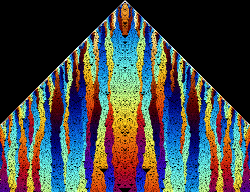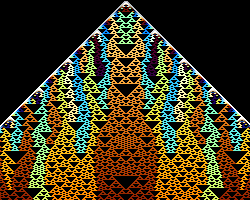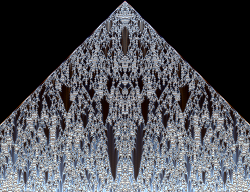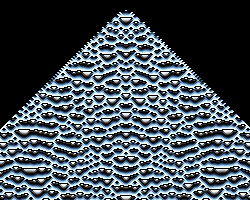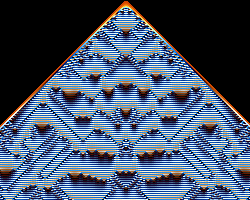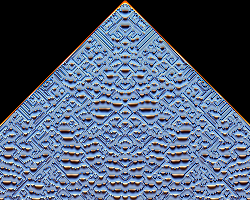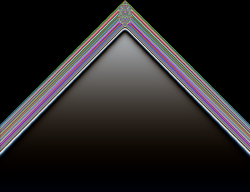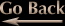Pascal's Triangle Fractals
Ivan Freyman, 03/25/2010

You may recall that Pascal's Triangle can be used to determine the coefficients of polynomial terms expanded from (x+y)^n. However, it can also be used to create pretty pictures when each number is used to determine the color of a pixel. Creating the Sierpinski triangle is the easiest: If the number is odd, make the pixel white, otherwise make it black. Even easier is to count in binary rather than base ten and light up only the pixels that correspond to 1's. The other pictures are a bit more involved but are all based on the idea of having a 'bail-out' value and somehow reducing the most recently computed Pascal's number if the bailout value is exceeded. One variation involves setting the number to zero or dividing it by a constant, i.e. 2 or 4.2. Another variation is to raise the number to a non-integer exponent between 0 and 1, or to take its square root. Next, regardless of whether the number bailed out or not, feed it into three sine functions, one for each color channel. Add some phase offsets and frequency coefficents to the sine functions to ensure the channels do not duplicate each other, and you'll get a colorful Pascal's Triangle. The results of my programming efforts are below.